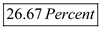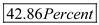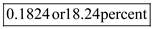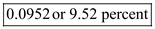## Quiz 12 : Investing in Stocks and BondsLooking for Finance Homework Help?# Quiz 12 : Investing in Stocks and Bonds

The following equation is used for calculating yield on investment, which is as follows:…… (1) a) To calculate yield on stock use the equation1, as follows: Substitute \$2 for ' Average Annual income ', \$60 for ' Future price ', \$30 for ' Current price ', and 3 years for ' Number of years 'Therefore, from the above calculations it can be concluded that, the option a can yieldb) To calculate yield on security use the equation 1, as follows: Substitute \$0 for ' Average Annual income ', \$100 for ' Future price ', \$40 for ' Current price ', and 2 years for ' Number of years 'Therefore, from the above calculations it can be concluded that, the option b can yieldc) The yield on the note as mentioned in the question is 5percent. Based on the above yield calculation the ranking of the investments are as follows: 1. Option b with 42.86 percent 2. Option a with 26.67 percent 3. Option c with 5 percent

The following formula is used for calculating yield on investment which is as follows:Substitute \$4 for ' Average Annual income ', \$115 for ' Future price ', \$75 for ' Current price ', and 3 years for ' Number of years 'Therefore, from the above calculations it can be concluded that,is the rate of return an investor can expect to earn on the investment.

The following formula is used for calculating yield on investment which is as follows:Substitute \$0 for ' Average Annual income ', \$125 for ' Future price ', \$85 for ' Current price ', and 4years for ' Number of years 'Therefore, from the above calculations it can be concluded that, the yield on stock iswhich is significantly lower than the expectation of Mr. S hence, he should not invest.